# begin_shape()#

Using the begin_shape() and end_shape() functions allow creating more complex forms.

## Examples#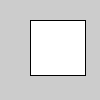def setup():
py5.begin_shape()
py5.vertex(30, 20)
py5.vertex(85, 20)
py5.vertex(85, 75)
py5.vertex(30, 75)
py5.end_shape(py5.CLOSE)def setup():
py5.begin_shape(py5.POINTS)
py5.vertex(30, 20)
py5.vertex(85, 20)
py5.vertex(85, 75)
py5.vertex(30, 75)
py5.end_shape()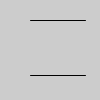def setup():
py5.begin_shape(py5.LINES)
py5.vertex(30, 20)
py5.vertex(85, 20)
py5.vertex(85, 75)
py5.vertex(30, 75)
py5.end_shape()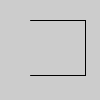def setup():
py5.no_fill()
py5.begin_shape()
py5.vertex(30, 20)
py5.vertex(85, 20)
py5.vertex(85, 75)
py5.vertex(30, 75)
py5.end_shape()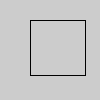def setup():
py5.no_fill()
py5.begin_shape()
py5.vertex(30, 20)
py5.vertex(85, 20)
py5.vertex(85, 75)
py5.vertex(30, 75)
py5.end_shape(py5.CLOSE)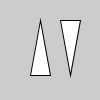def setup():
py5.begin_shape(py5.TRIANGLES)
py5.vertex(30, 75)
py5.vertex(40, 20)
py5.vertex(50, 75)
py5.vertex(60, 20)
py5.vertex(70, 75)
py5.vertex(80, 20)
py5.end_shape()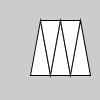def setup():
py5.begin_shape(py5.TRIANGLE_STRIP)
py5.vertex(30, 75)
py5.vertex(40, 20)
py5.vertex(50, 75)
py5.vertex(60, 20)
py5.vertex(70, 75)
py5.vertex(80, 20)
py5.vertex(90, 75)
py5.end_shape()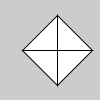def setup():
py5.begin_shape(py5.TRIANGLE_FAN)
py5.vertex(57.5, 50)
py5.vertex(57.5, 15)
py5.vertex(92, 50)
py5.vertex(57.5, 85)
py5.vertex(22, 50)
py5.vertex(57.5, 15)
py5.end_shape()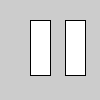def setup():
py5.vertex(30, 20)
py5.vertex(30, 75)
py5.vertex(50, 75)
py5.vertex(50, 20)
py5.vertex(65, 20)
py5.vertex(65, 75)
py5.vertex(85, 75)
py5.vertex(85, 20)
py5.end_shape()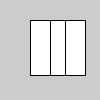def setup():
py5.vertex(30, 20)
py5.vertex(30, 75)
py5.vertex(50, 20)
py5.vertex(50, 75)
py5.vertex(65, 20)
py5.vertex(65, 75)
py5.vertex(85, 20)
py5.vertex(85, 75)
py5.end_shape()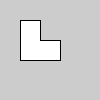def setup():
py5.begin_shape()
py5.vertex(20, 20)
py5.vertex(40, 20)
py5.vertex(40, 40)
py5.vertex(60, 40)
py5.vertex(60, 60)
py5.vertex(20, 60)
py5.end_shape(py5.CLOSE)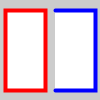def setup():
py5.translate(25, 50)
py5.stroke_weight(4)
py5.stroke("#F00")
with py5.begin_closed_shape():
py5.vertex(-20, -40)
py5.vertex(20, -40)
py5.vertex(20, 40)
py5.vertex(-20, 40)

py5.translate(50, 0)
py5.stroke("#00F")
with py5.begin_shape():
py5.vertex(-20, -40)
py5.vertex(20, -40)
py5.vertex(20, 40)
py5.vertex(-20, 40)


## Description#

Using the begin_shape() and end_shape() functions allow creating more complex forms. begin_shape() begins recording vertices for a shape and end_shape() stops recording. The value of the kind parameter tells it which types of shapes to create from the provided vertices. With no mode specified, the shape can be any irregular polygon. The parameters available for begin_shape() are POINTS, LINES, TRIANGLES, TRIANGLE_FAN, TRIANGLE_STRIP, QUADS, and QUAD_STRIP. After calling the begin_shape() function, a series of vertex() commands must follow. To stop drawing the shape, call end_shape(). The vertex() function with two parameters specifies a position in 2D and the vertex() function with three parameters specifies a position in 3D. Each shape will be outlined with the current stroke color and filled with the fill color.

Transformations such as translate(), rotate(), and scale() do not work within begin_shape(). It is also not possible to use other shapes, such as ellipse() or rect() within begin_shape().

The P2D and P3D renderers allow stroke() and fill() to be altered on a per-vertex basis, but the default renderer does not. Settings such as stroke_weight(), stroke_cap(), and stroke_join() cannot be changed while inside a begin_shape() & end_shape() block with any renderer.

This method can be used as a context manager to ensure that end_shape() always gets called, as shown in the last example. Use begin_closed_shape() to create a context manager that will pass the CLOSE parameter to end_shape(), closing the shape.

Underlying Processing method: beginShape

## Signatures#

begin_shape() -> None

begin_shape(
kind: int,  # Either POINTS, LINES, TRIANGLES, TRIANGLE_FAN, TRIANGLE_STRIP, QUADS, or QUAD_STRIP
/,
) -> None


Updated on March 06, 2023 02:49:26am UTC